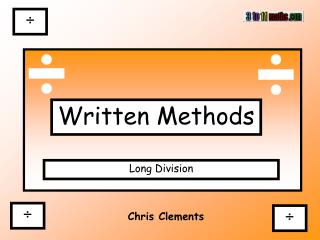DownloadDownload PresentationChris Clements

# Chris Clements

Download Presentation## Chris Clements

- - - - - - - - - - - - - - - - - - - - - - - - - - - E N D - - - - - - - - - - - - - - - - - - - - - - - - - - -
##### Presentation Transcript

1. ÷ Written Methods Long Division ÷ ÷ Chris Clements

2. <date> Learning Objective: LO: I can understand and use long division.

3. How to do Long Division: 504 ÷ 21 = Before you begin to solve an equation, using a long written method, it is important to make an approximation first. If we round 504 to 500 and 21 to 20 we make an equation we can do mentally. We can use this to check the answer at the end. 500 ÷ 20 = 25

4. Set the sum out correctly Start with the largest place holder in this case it will be the hundreds column. 0 2 4 5 ÷ 21 not possible So put a 0 above the hundreds column - 4 2 Carry the 5s digit over to the Tens column! 8 4 4 50 ÷ 21 = - 8 21 x 2 = 42 84 ÷ 21 = 4 0 50 – 42 = 8 No remainders

5. Set the sum out correctly 0 2 4 The answer is 24; check using approximation. If the approximation is nowhere near your answer. You will need to go back and check each of your calculations for mistakes. 500 ÷ 20 = 25

6. 1) Make an approximation (Use to check answer) 2) Carry out calculation (see below) 3) Check your answer Long Division Steps to success 0 2 4 2 - 4 8 4 - 8 4 0 No remainders

7. Try this equation; 848 ÷ 16 Approximation 800 ÷ 16 = 50 Start with the largest place holder in this case it will be the hundreds column. 0 5 3 8 ÷ 16 not possible So put a 0 above the hundreds column - 8 0 Carry the 8s digit over to the Tens column! 4 8 8 84 ÷ 16 = - 4 16 x 5 = 80 48 ÷ 16 = 3 0 84 – 80 = 4 No remainders

8. Set the sum out correctly 0 5 3 The answer is 53; check using approximation. If the approximation is nowhere near your answer. You will need to go back and check each of your calculations for mistakes. 800 ÷ 16 = 50

9. Activity <type here>

10. Plenary May saved her pocket money for a number of weeks so that she could buy her favourite packs of stickers. She saved £6 and stickers cost 18p a pack. How many packs could you afford to buy? 0 3 3 - 5 4 6 0 4 - 5 6 stickers in each pack 6 How many stickers did she buy? 33 x 6 = 198 6p How much change did she get?